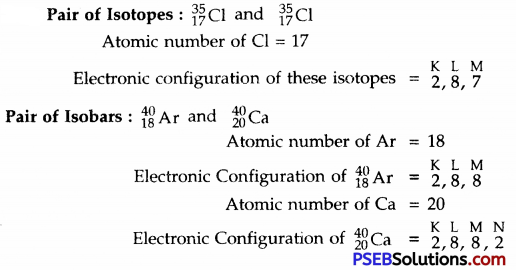# PSEB 9th Class Science Solutions Chapter 4 Structure of the Atom

Punjab State Board PSEB 9th Class Science Book Solutions Chapter 4 Structure of the Atom Textbook Exercise Questions and Answers.

## PSEB Solutions for Class 9 Science Chapter 4 Structure of the Atom

PSEB 9th Class Science Guide Structure of the Atom Textbook Questions and Answers

Question 1.
Compare the properties of electron, proton and neutron.

 Particle Charge (e) Mass (m) Charge/Mass (e/m) Electron – 1.6022 × 1019C 9.109 × 1028g – 1.76 × 108 Proton + 1.6022 × 10-19C 1.672 × 10-24g + 9.58 × 104 Neutron Zero 1.675 × 10-24g ZeroQuestion 2.
What are the limitations of J.J. Thomson model of atom?
Limitations of J.J. Thomson model of atom

• It could not explain stability of the atom.
• It could not explain hydrogen spectrum.

Question 3.
What are the limitations of Rutherford’s model of atom?
Limitations of Rutherford’s model of atom

• It can’t explain stability of the atom.
• It can’t explain hydrogen spectrum.

Question 4.
Describe Bohr’s model of atom.
The main points of Bohr’s model of atom are:
1. An atom has three types of particles called fundamental particles. These are electrons, protons and neutrons.
2. The protons and neutrons are present in the nucleus, present in the centre. The electrons are present around the nucleus and at very large distances from it. There is a large vacant space between the nucleus and the electrons.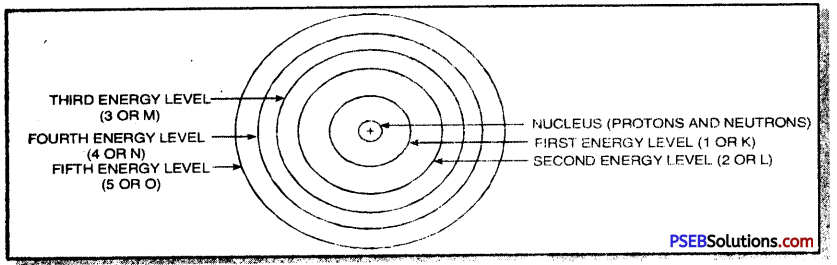3. An atom is electrically neutral as the number of protons is equal to the number of electrons.
4. The electrons are revolving around the nucleus in fixed circular paths which are called energy levels or energy shells or orbits. The energy levels or energy shells can be counted in two ways 1, 2, 3, 4, 5, 6 or as K, L, M, N, O, P. The counting starts from the centre outwards.
5. The various energy levels are arranged in order of increasing energy. The order of energy is 1 < 2 < 3 < 4 < 5 or K < L < M < N < O ………..
6. The energy of an electron in an atom is quantised.
7. There is no change in the energy of the electrons as long as they keep on revolving in the same energy level.
8. The angular momentum of an electron in an atom is quantised.
9. The change in energy can take place only when the electron jumps from one energy level to the other. If the electron gains energy from outside, it jumps from lower energy to the higher energy level. If the electron jumps from higher energy level to the lower energy level, it loses energy.Question 5.
Compare all the proposed models of atom given in the chapter 3 of the text.
Comparing Thomson’s model, Rutherford’s model and Bohr’s model of atom.
A. Thomson’s model of atom.
1. According to Thomson, an atom may be regarded, a uniform sphere of positive electricity (protons) in which negatively charged electrons are embedded like the seeds in a watermelon.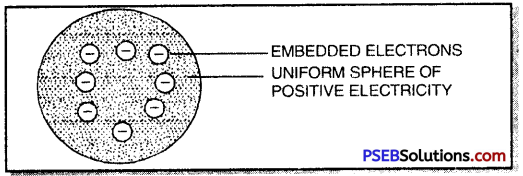2. The total positive charge is equal to the total negative charge on all the electrons so that atoms on the whole is electrically neutral.

B. Rutherford’s nuclear model of atom.
The main features of Rutherford’s nuclear model of atom are:

1. Atom has a dense, heavy, positively charged central part called nucleus.
2. The electrons are present at very large distances from the nucleus.
3. The total positive charge on the nucleus is equal to the total ~ve charge on all the electrons so that atom on the whole is electrically neutral.
4. The electrons are revolving around the nucleus so that attractive force of the nucleus is balanced by the centrifugal force (just like planets revolve around the sun).
5. It is different from Thomson’s model, because in Thomson’s model of atom, the total mass, positive charge and electrons are uniformly distributed

C. Bohr’s model of atom. Described in given above.

Question 6.
Summarize the rules for writing of distribution of electrons in various shells for the first eighteen elements.
The various rules for writing the electronic configuration of first elements are:
1. The energy levels or energy shells are filled in order of increasing energies. The electrons first enter the K shell (n =

1. which is closest to the nucleus. This is followed by L shell (n = 2), M shell (n = 3) and so on. Here V represents the number of the shell.
2. The maximum number of electrons in any shell is given as 2n2 (n = No. of shells).
3. The outermost energy shell cannot have more than 8 electrons. The next inner shell called penultimate shell cannot have more than 18 electrons.
4. It is not necessary that an energy level or shell is fully filled before the filling in the next energy level starts. In fact filling of electrons in a new shell starts when any shell contains 8 electrons.Question 7.
Define valency by taking examples of silicon and oxygen.
Valency: It is the combining capacity of an atom of the element and is numerically equal to the number of electrons present in the valence shell of an atom (if the no. of electrons is 1 to 4) or eight minus the number of electrons present in the valence shell of an atom (if the no. of electrons is more than 4).
e.g. Silicon (14Si) has electronic configuration = 2, 8, 4
∴ its valency = 4 (No. of electrons in its valence shell)
Oxygen (8O) has electronic configuration = 2, 6
∴ its valency = 8 – 6 = 2 (8 – No. of electrons in the valence shell)

Question 8.
Explain with examples

1. Atomic number
2. Mass number
3. Isotopes
4. Isobars. Give any two uses of isotopes.

1. Atomic number. It is the number of protons present in the nucleus of an atom of an element.
2. Mass number. It is the sum of total number of protons and neutrons present in the nucleus of an atom.
3. Isotopes. These are the atoms of the same element having same atomic number but different mass numbers.
4. Isobars. These are the atoms of different elements having same mass number but different atomic numbers.

Uses of isotopes:

1. $${ }_{92}^{235} \mathrm{U}$$ isotope of uranium is used as a fuel in nuclear reaction.
2. $${ }_{27}^{60} \mathrm{Co}$$ isotope of cobalt is used in the treatment of cancer.
3. $${ }_{53}^{131} \mathrm{I}$$ isotope of iodine is used in the treatment of goitre.

Question 9.
Na+ has completely filled K and L shells. Explain.
Na has atomic number =11
∴ Na atom has electrons =11
Na+ ion has electrons = 11 – 1 = 10
The electronic configuration of Na+ = 2, 8
∴ In Na+, K and L-shells are completely filled.Question 10.
If bromine atom is in the form of say two isotopes 35 Br (49.7%) and 35 Br (50.3%), then calculate the average mass of bromine atom.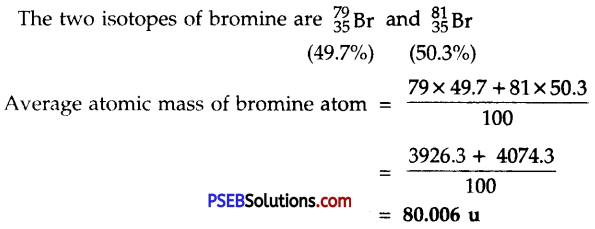Question 11.
The average atomic mass of a sample of an element X is 16.2 u, what are the percentages of isotopes g X and “X in the sample?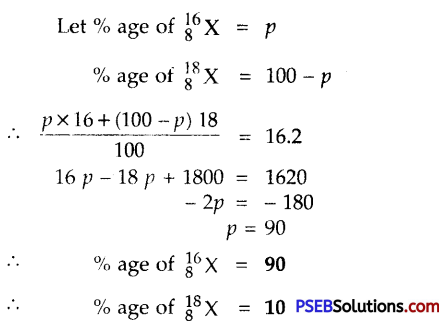Question 12.
If Z = 3, what would be the valency of the element? Also, name the element.
Z = 3

• Atomic no. of element = 3
• No. of electrons in one atom of the element = 3
• Atomic no. of the element (Z) = 3
• Its electronic configuration = 2, 1
• Hence its valency = 1 (No. of electrons in the valence shell.)
• The name of the element = Lithium.

Question 13.
Composition of the nuclei of two atomic species X and Y are given as under:Give the mass numbers of X and Y. What is the relation between the two species?

• Mass no. of X = 6 + 6 = 12
• Mass no. of Y = 6 + 8 = 14
• The two species are isotopes because they have same atomic number but different mass numbers.Question 14.
For the following statements write T for True and F for False.
(a) J.J. Thomson proposed that the nucleus of an atom contains only nucleons.
(b) A neutron is formed by an electron and a proton combining together. Therefore, it is neutral.
(c) The mass of an electron is about 1/2000 times that of proton.
(d) Isotope of iodine is used for making tincture iodine, which is used as a medicine.
(a) F
(b) F
(c) T
(d) F.

Put tick (✓) against correct choice and cross (×) against wrong choice in questions 15, 16 and 17

Question 15.
Rutherford’s alpha-particle scattering experiment was responsible for the discovery of :
(a) Atomic Nucleus
(b) Electron
(c) Proton
(d) Neutron
(a) Atomic Nucleus (✓)
(b) Electron (×)
(c) Proton (×)
(d) Neutron (×)

Question 16.
Isotopes of an element have:
(a) the same physical properties
(b) different chemical properties
(c) different number of neutrons
(d) different atomic numbers.
(a) the same phvsicul properties (×)
(b) different chemical properties (×)
(c) different number of neutrons (✓)
(d) different atomic numbers (×)

Question 17.
Number of valence electrons in Cl+ ion are:
(a) 16
(b) 8
(c) 17
(d) 18
(a) 16 (×)
(b) 8 (×)
(c) 17 (×)
(d) 18 (×).Question 18.
Which one of the following is a correct electronic configuration of sodium?
(a) 2, 8
(b) 8, 2, 1
(c) 2, 1, 8
(d) 2, 8, 1.
(d) 2, 8, 1.

Question 19.
Complete the following table:

 Atomic Number Mass Number Number of Neutrons Number of Protons Number of Electrons Name of the Atomic Species 9 – 10 ~ – – 16 32 – – – Sulphur – 24 – 12 – – – 2 – 1 – – – 1 0 1 0 –

 Atomic Number Mass Number Number of Neutrons Number of protons Number of Electrons Name of the Atomic Species 9 19 10 9 9 Fluorine 16 32 16 16 16 Sulphur 12 24 12 12 12 Magnesium 1 2 1 1 1 Deuterium 1 1 0 1 0 Protium

Science Guide for Class 9 PSEB Structure of the Atom InText Questions and Answers

Question 1.
What are canal rays?
A beam of rays or stream of particles which travel in a direction away from anode, towards cathode, when any gas taken in a discharge tube is subjected to the action of high voltage under low pressure are called canal rays.Question 2.
If an atom contains one electron and one proton, will it carry any charge or not?
No, it will not carry any charge.

Question 3.
On the basis of Thomson’s model of an atom explain how the atom is neutral as a whole.
Thomson’s model of atom.
1. According to Thomson, an atom may be regarded as a uniform sphere of positive electricity (protons) in which negatively charged electrons are embedded like the seeds in a watermelon.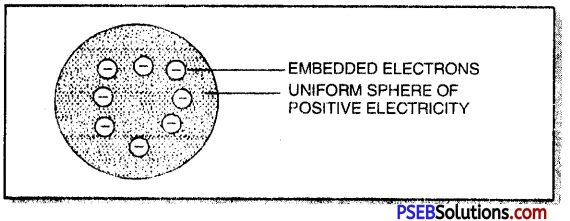2. The total positive charge is equal to the total negative charge on all the electrons so that atoms on the whole is electrically neutral.

Question 4.
On the basis of Rutherford’s model of an atom which sub-atomic particle is present in the molecule of an atom?
Proton.

Question 5.
Draw a sketch of Bohr’s model of an atom with three shells.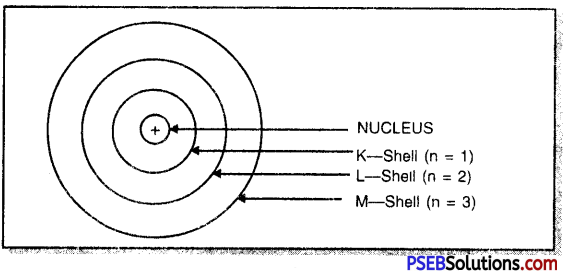Question 6.
What do you think would be the observation if the ∝-particle scattering experiment is carried out using a foil of metal other than gold?
Almost all the ∝-particles will pass undeflected and hardly any a-particle is deflected.Question 7.
Name the three sub-atomic particles of an atom.
These are:
(a) Electron
(b) Proton and
(c) Neutron

Question 8.
Helium atom has an atomic mass of 4 u and two protons in its nucleus. How many neutrons does it have?
Atomic mass of Helium = 4 u No. of protons = 2
∴ No. of neutrons = 4 – 2 = 2

Question 9.
Write the distribution of electrons in carbon and sodium atoms.

 Element At. No. Electronic Configuration K L M Carbon 6 2 4 1 Sodium 11 2 8 1

Question 10.
If K and L shell of an atom are full then what would be the total number of electrons in it?

1. No. of electrons in K-shell = 2
2. No. of electrons in L-shell = 8
3. Total no. of electrons = 2 + 8 = 10

Question 11.
How will you find the valency of chlorine, sulphur and magnesium?
Chlorine has the electronic configuration = 2, 8, 7
∴ Valency of chlorine = 8 – 7 = 1
Sulphur has the electronic configuration = 2, 8, 6
∴ Valency of sulphur = 8 – 6 = 2
Magnesium has the electronic configuration = 2, 8 ,2
∴ Valency of magnesium = 2Question 12.
If number of electrons in an atom are 8 and number of protons are also 8, then ;
1. What is the atomic number of the atom?
2. What is the charge on the atom?
1. No. of protons = 8
∴ Atomic no. of the element = 8
2. Zero.

Question 13.
With the help of table 4.1 (given in Text Book) find out the mass number of oxygen and sulphur atom.

• Mass number of oxygen = 8 + 8 = 16
• Mass number of sulphur = 16 + 16 = 32

Question 14.
For the symbols H, D and T tabulate three fundamental particles found in each of them.